# International Economics Study Set 8

## Quiz 14 : Money, Interest Rates, and Exchange RatesLooking for Economics Homework Help?

## Quiz 14 :Money, Interest Rates, and Exchange Rates

Question TypeSuppose there is a reduction in aggregate real money demand, that is, a negative shift in the aggregate real money demand function. Trace the short- and long-run effects on the exchange rate, interest rate, and price level.
Free
Essay

To trace the short-run and long-run effects of a reduction in the aggregate real money demand on the exchange rate, interest rate, and price level, one must link the money market to the foreign exchange market.
The money market establishes the equilibrium interest rate by satisfying the condition: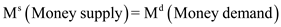Or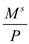(Real money supply)=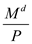(Real money demand)Alternatively,=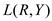.
On the other hand, the foreign exchange rate equilibrium is established by the interest parity condition between the domestic currency and the foreign currency.
The simultaneous equilibrium in the money market and exchange market is shown below: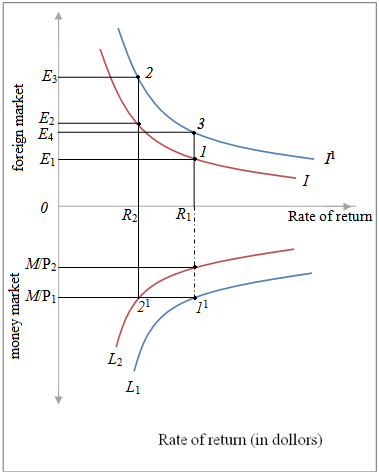Both asset markets are in equilibrium at the interest rate R 1 and exchange rate E 1 ; at these values, real money supply equals real money demand (point 1')and the interest parity condition holds (point 1)Now, when aggregate real money demand reduces, it shifts the real money demand schedule towards the left from L 1 to L 2. In the short run, a decrease in the real money demand will push the interest rate down from R 1 to R 2 ; also the price level, which will have immediate repercussions in the exchange market.
In an open economy, where interest parity between countries must be preserved, the exchange rate will increase (currency depreciation)to create the expectation that it will fall faster in the future.
It should be noted that, the depreciation of the currency would depend on whether the contraction in money demand is deemed permanent or temporary.
If the reduction in money demand is temporary, the currency will depreciate from E 1 to E 2 and if the reduction is permanent, it will depreciate to E 3.
However, in the long run, there is a permanent reduction in money demand.
As a result, the price level rises so that real money supply adjusts to become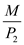in order to match the fall in real money demand, making the interest rate equal to its initial value.
In addition, the domestic currency will depreciate as exchange rate moves to E 4 in proportion to the fall in real money demand.
To sum up, a reduction in the aggregate real money demand will lead to a new equilibrium point 2' in the money market, simultaneous short run equilibrium at point 2, and long run equilibrium at point 3 in the foreign exchange market.

TagsHow would you expect a fall in a country's population to alter its aggregate money demand function? Would it matter if the fall in population were due to a fall in the number of households or to a fall in the size of the average household?
Free
Essay

Aggregate money demand refers to the total of all expenditures in the economy (which equals the GDP). Several factors cause changes in the aggregate money demand.
One of the important factors that influence aggregate money demand is the population of the country. If a country's population falls, various components of expenditure falls. A reducing population will have low consumption expenditure. Therefore, aggregate money demand falls.
Now, if the fall in population is due to a fall in the number of households, the reducing effect on the aggregate money demand will be more.
It reveals the fact that, there is a direct relationship between trends in population and consumption expenditures; thus with the aggregate money demand.
However, if the fall in population is due to the fall in the size of the average household, the reducing effect on the aggregate money demand will be less.
A fall in the size of the average household implies the variation in the composition of a household; that is a household consists of more middle aged people and older people whose marginal propensity ( MPC )to consume is higher and fewer children whose MPC is low.
Moreover, such a small size of average household does not reduce the income level of the household and thus cannot affect the consumption expenditure.
Therefore, a fall in the number of households affects the aggregate money demand more than the fall in the size of the average household.

TagsThe velocity of money, V , is defined as the ratio of real GNP to real money holdings, V = Y /( M / P ) in this chapter's notation. Use equation to derive an expression for velocity and explain how velocity varies with changes in R and in Y. (Hint: The effect of output changes on V depends on the elasticity of aggregate money demand with respect to real output, which economists believe to be less than unity.) What is the relationship between velocity and the exchange rate?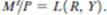Free
Essay

Velocity of money refers to the number of times that a unit of money spent on the total value of goods and services produced per year. It is defined as the ratio of real GNP to real money holdings and is given as:…… (1)Now, equation (15-4)of the chapter is as follows: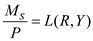…… (2)Substituting the value for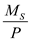in equation (1), we can derive an expression for velocity as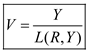…… (3)The money demand is negatively correlated with the interest rate, an increase in interest rate will increase the opportunity cost of holding money. Thus, aggregate demand for moneyfalls.
It is revealed from the above expression that; velocity and interest rate move in the same direction.
An increase in the value of R will result in a decrease in the value for liquidity preferenceand thus an increase in the velocity of money.
An increase in the value of Y , will result in a smaller increase in the value of liquidity preference;. The increase inis small because the elasticity of aggregate money demand is less than one; with respect to real output.
Thus, an increase in Y , results in an increase in the fraction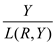.
The value of velocity V increases, with an increase in interest rate or with an increase in income. Since the increase in velocity is associated with an increase in income as well as an increase in interest rates; the increase in velocity is said to be associated with an appreciation of the exchange rate.
Now, to recall, according to interest parity condition, an increase in interest rate leads to fall in the exchange rate; that is the domestic currency will appreciate.
As an increase in interest rate causes the money velocity to increase as per the equation (3)and since that increase in interest rate causes exchange rate to fall, an increase in money velocity will cause exchange rate to fall; that is appreciation of the domestic currency.
Therefore, the relationship between velocity of money and the exchange rate is inverse i.e. greater is the velocity of money, lower is the exchange rate; resulting in the appreciation of the currency.

TagsWhat is the short-run effect on the exchange rate of an increase in domestic real GNP, given expectations about future exchange rates?
Essay
TagsDoes our discussion of money's usefulness as a medium of exchange and unit of account suggest reasons why some currencies become vehicle currencies for foreign exchange transactions? (The concept of a vehicle currency was discussed in Chapter 3.)
Essay
TagsIf a currency reform has no effects on the economy's real variables, why do governments typically institute currency reforms in connection with broader programs aimed at halting runaway inflation? (There are many instances in addition to the Turkish case mentioned in the text. Other examples include Israel's switch from the pound to the shekel, Argentina's switches from the peso to the austral and back to the peso, and Brazil's switches from the cruzeiro to the cruzado, from the cruzado to the cruzeiro, from the cruzeiro to the cruzeiro real, and from the cruzeiro real to the real, the current currency, which was introduced in 1994.)
Essay
TagsIn 1984 and 1985, the small Latin American country of Bolivia experienced hyperinflation. Below are some key macroeconomic data from those years: Macroeconomic Data for Bolivia, April 1984-October 1985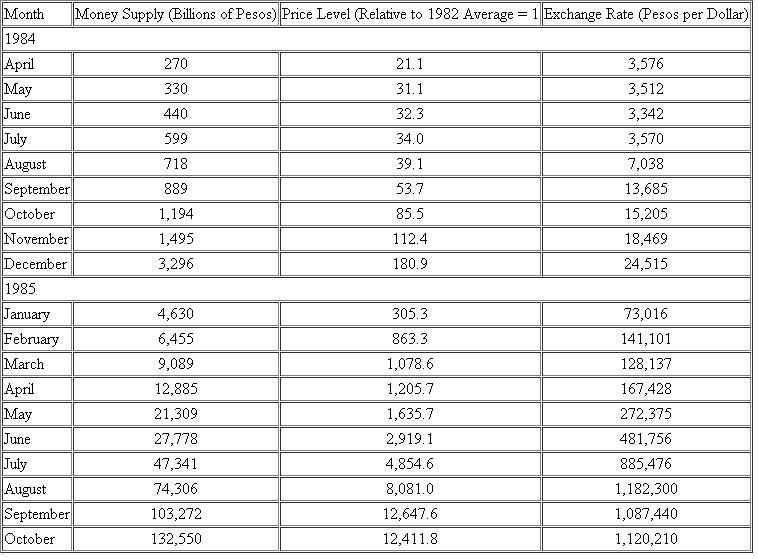Source: Juan-Antonio Morales, "Inflation Stabilization in Bolivia," in Michael Bruno et al., eds., Inflation Stabilization: The Experience of Argentina, Brazil, Bolivia, and Mexico. Cambridge, MA: MIT Press, 1988, table 7A-1. Money supply is M1. a. Do the money supply, price level, and exchange rate against the U.S. dollar move broadly as you would expect? Explain. b. Calculate the percent changes in the general price level and price of the dollar between April 1984 and July 1985. How do these compare to each other and to the percent increase in the money supply? Can you explain the results? (Hint: Refer back to the discussion of the velocity of money in question 3.) c. The Bolivian government introduced a dramatic stabilization plan near the end of August 1985. Looking at the price levels and exchange rates for the following two months, do you think it was successful? In light of your answer, explain why the money supply increased by a large amount between September and October 1985.
Essay
TagsBelow is a table of some inflation targeting countries and the years in which they adopted the practice: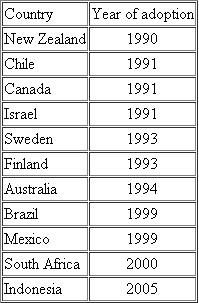Go to the International Monetary Fund's most recent World Economic Outlook database (accessible directly or through www.imf.org) and collect the annual inflation rate series PCPIEPCH for these countries, starting in 1980. Then graph the data for each country using Excel or some other data analysis package. Just looking at the data, does inflation appear to behave differently after the adoption of inflation targeting?
Essay
TagsIn our discussion of short-run exchange rate overshooting, we assumed real output was given. Assume instead that an increase in the money supply raises real output in the short run (an assumption that will be justified in Chapter 6). How does this affect the extent to which the exchange rate overshoots when the money supply first increases? Is it likely that the exchange rate undershoots? (Hint: In Figure, allow the aggregate real money demand schedule to shift in response to the increase in output.)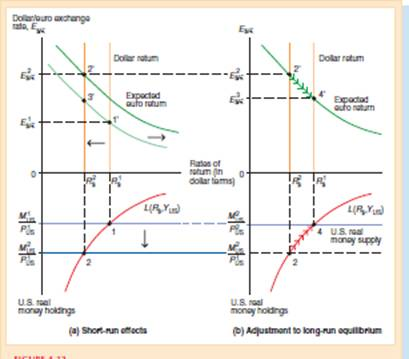Short-Run and Long-Run Effects of an Increase in the U.S. Money Supply (Given Real Output, Y ) (a) Short-run adjustment of the asset markets. (b) How the interest rate, price level, and exchange rat move over time as the economy approaches its long-run equilibrium.
Essay
TagsFigure shows that Japan's short-term interest rates have had periods during which they are near or equal to zero. Is the fact that the yen interest rates shown never drop below zero a coincidence, or can you think of some reason why interest rates might be bounded below by zero?Short-Run and Long-Run Effects of an Increase in the U.S. Money Supply (Given Real Output, Y ) (a) Short-run adjustment of the asset markets. (b) How the interest rate, price level, and exchange rat move over time as the economy approaches its long-run equilibrium.
Essay
TagsHow might a zero interest rate complicate the task of monetary policy? (Hint: At a zero rate of interest, there is no advantage in switching from money to bonds.)
EssayAs we observed in this chapter, central banks, rather than purposefully setting the level of the money supply, usually set a target level for a short-term interest rate by standing ready to lend or borrow whatever money people wish to trade at that interest rate. (When people need more money for a reason other than a change in the interest rate, the money supply therefore expands, and it contracts when they wish to hold less.) a. Describe the problems that might arise if a central bank sets monetary policy by holding the market interest rate constant. (First, consider the flexible-price case, and ask yourself if you can find a unique equilibrium price level when the central bank simply gives people all the money they wish to hold at the pegged interest rate. Then consider the sticky-price case.) b. Does the situation change if the central bank raises the interest rate when prices are high, according to a formula such as R - R 0 = a ( P - P 0 ), where a is a positive constant and P 0 a target price level? c. Suppose the central bank's policy rule is R - R 0 = a ( P - P 0 ) + u , where u is a random movement in the policy interest rate. In the overshooting model shown in Figure, describe how the economy would adjust to a permanent one-time unexpected fall in the random factor u , and say why. You can interpret the fall in u as an interest rate cut by the central bank, and therefore as an expansionary monetary action. Compare your story with the one depicted in Figure.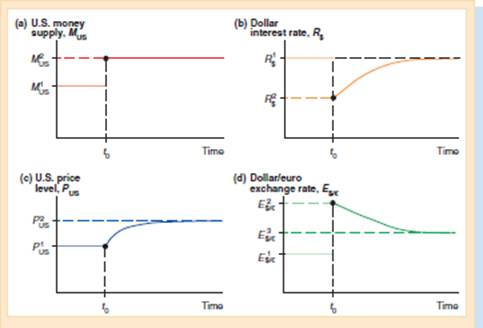Time Paths of U.S. Economic Variables after a Permanent Increase in the U.S. Money Supply After the money supply at t 0 in pane (a), the interest rat (in pane (b)), price level (in pane (c)), and exchange rate (in pane (d)) move as shown toward their long-run levels. As indicted in panel (d) by the initial jump from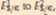the exchange rate over shoots in the short run before settling down to its long-run level,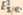.